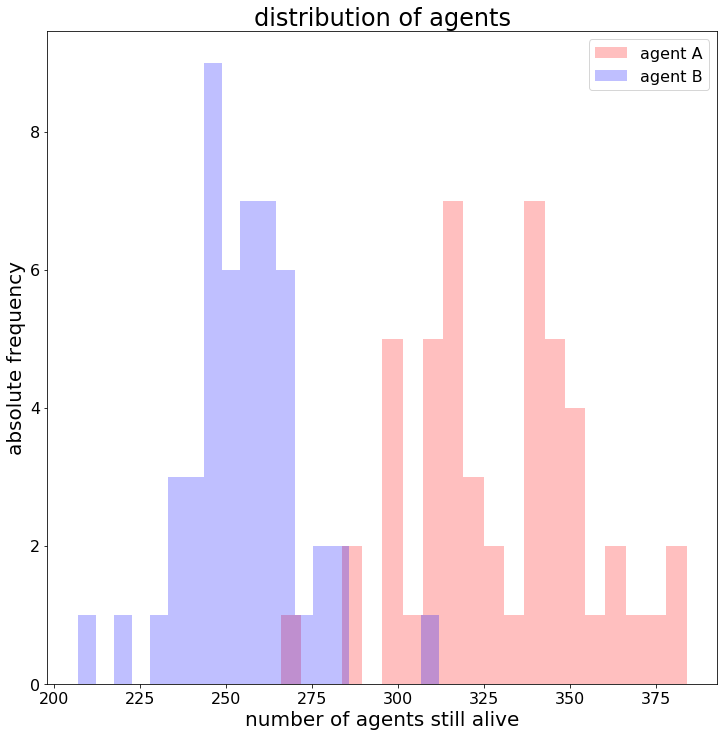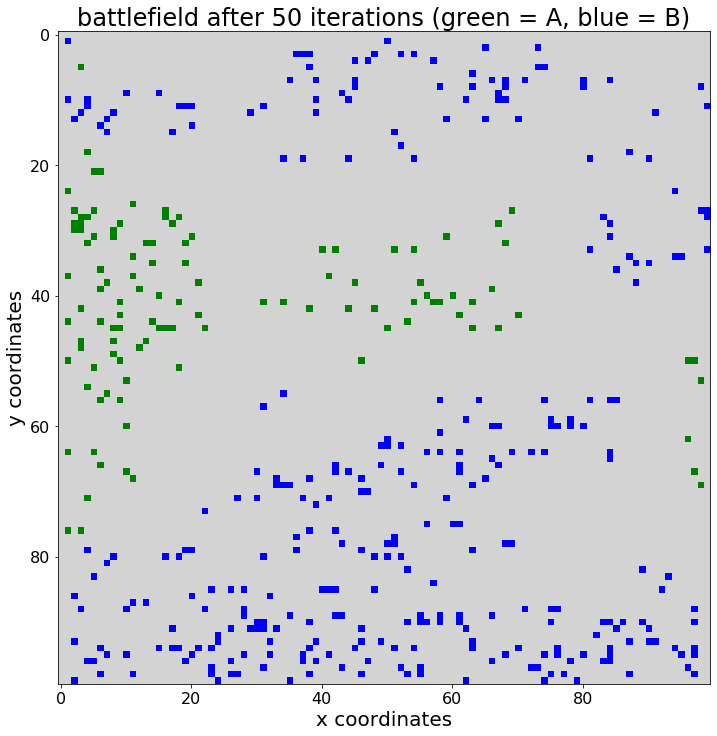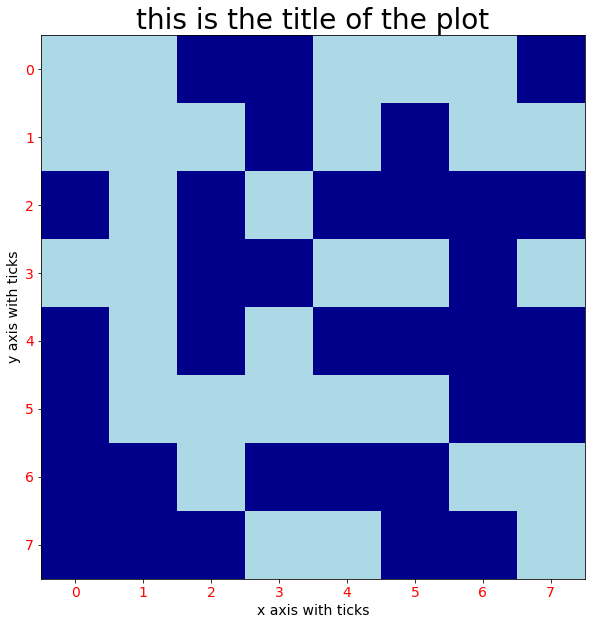# Agent-based simulation

## Developing an agend-based framework for simulation models in PythonIn a previous post I constructed a simple agent-based simulation model, containing groups of agents that can be located on a battlefield grid. The model was coded in Python, using matplotlib for visualization. I went on to conduct a simple […]

## A simple agent-based simulation run, visualized using Matplotlib in PythonIn a previous post I constructed a simple agent-based simulation model, containing groups of agents that can be located on a battlefield grid. The model was coded in Python, using matplotlib for visualization. The agents were modelled as a class, […]

## Developing a simple agent-based simulation model in PythonIn a previous post I demonstrated how to visualize a 2D grid, using matplotlib and pyplot in Python (post titled “Visualizing 2D grids and arrays using matplotlib in Python”). That post was meant as first introduction on how to visualize […]

## Visualizing 2D grids and arrays using matplotlib in PythonA 2D grid array plot can be a valuable visualization tool, e.g. in the area of agent-based simulation. In this post I want to give a brief tutorial in how you can visualize a 2D grid array, using matplotlib in […]